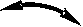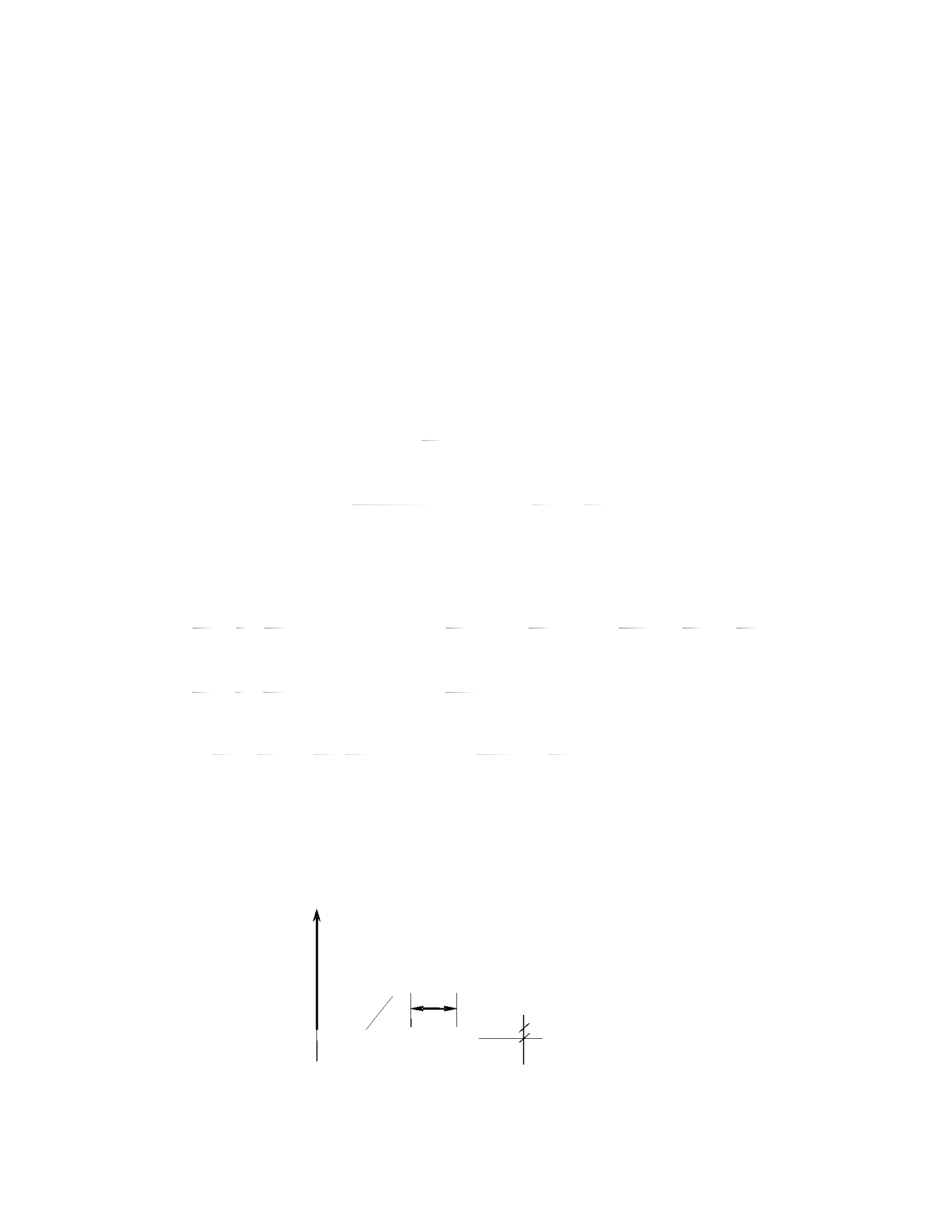Vietnam Journal of Mechanics, VAST, Vol.30, No.3 (2008), pp. 133–141
A NEW APPROACH FOR INVESTIGATING
CORRUGATED LAMINATED COMPOSITE
PLATES OF WAVE FORM
Dao Huy Bich
Vietnam National University, Hanoi
Khuc Van Phu
Abstract. Corrugated plates of wave form made of isotropic elastic material were con-
sidered as flat orthotropic plates with corresponding orthotropic constants determined
empirically by the Seydel’s technique. In some recent researches the extension of this
technique was given for corrugated laminated composite plates.
In the present paper a new approach for investigating corrugated composite plate of
wave form is proposed, regarding this plates as a combination of parts of shallow cylindri-
cal shells with alternative curvatures. It reduces to no use of Seydel’s empirical formulas
and sufficiently apply to composite plates. Based on this approach governing equations of
corrugated laminated composite plate of wave form are developed and application to the
non-linear stability problem of this plate is considered. Obtained results are compared
with those of Seydel’s technique.
1.
INTRODUCTION
Corrugated plates of wave form made of isotropic elastic material were considered as
flat orthotropic plates with corresponding orthotropic constants determined empirically
by Seydel’s technique. This approach was acceptable to solve many bending and stability
problems of corrugated isotropic elastic plates in practice [4, 5, 7]. However, the analysis
of corrugated laminated composite plates has received comparitively little attention.
In  the authors developed the Seydel’s technique to the bending problems of cor-
rugated laminated composite plates and cylindrical shells. In the stability problem of
corrugated laminated composite plates  besides bending stiffnesses to be extended it is
necessary to formulate extensional stiffnesses and to define more exactly the strain expres-
sion by including the curvature of middle surface of corrugated plate. But the extension of
Seydel’s technique to corrugated composite plates meets with difficulties in determination
of corresponding constants and experimental verificationof these constants. Consequently,
obtained calculation may not describe the real state of corrugated laminated plates.
In other hand, nowaday corrugated laminated composite plates and cylindrical shells
arewidelyused andtheirstaticanddynamicproblemswithgeometricalnon-linearityareof
significantpractical interest, particularlystabilityand post-buckling behavior ofcomposite
plates and shells is more important. Therefore it needs more accuracy in investigating
corrugated composite plates and shells.
In order to eliminate this restriction, a new approach for investigating corrugated
composite plates of wave form is proposed naturally in the present paper, regarding thisπx
3
2
l l
/
 
∂u
1 ∂w
∂w
∂ ∂ w ∂u ∂k
ε =
+ ,
2
∂ w
∂v ∂w ∂w
∂u
2
∂ w ∂u
134
Dao Huy Bich, Khuc Van Phu
plate as a combination of shallow cylindrical shell parts with alternative curvatures. It
reduces to no use of Seydel’s empirical formulas and can sufficiently apply not only to an
isotropic elastic corrugated plate, but to a composite corrugated plate as well.
Based on this approach governing equations of a corrugated laminated composite plate
of wave form are developed and an application to the non-linear stability problem of this
plate is considered. Obtained results are compared with those of Seydel’s technique.
2.
GOVERNING EQUATIONS
Consider a rectangular symmetrically laminated composite corrugated plate in the
form of a sine wave(see Fig. 1), each layer of which is an unidirectional composite material.
Suppose the portion of cross-section line of a corrugated plate in the plane (x,z) has the
form of a sine wave
z = H sin l with H << l,
so that the alternative curvature of cross-section line is
k = z00 z00 = H.π2.sin πx.
(1 +z02) 2
(1)
Based on the new approach the non-linear strain-displacement relationships in the
middle surface and the changes of curvature and twist of a such corrugated plate now can
be written in the form
2
εx = ∂x + 2 ∂x kw,
   2 
χx = ∂x k.u+ ∂x = ∂x2 + k∂x +u∂x ,
∂v 1 ∂w2
y ∂y 2 ∂y
χy = ∂y2 ,
 
γxy = ∂y + ∂x + ∂x. ∂y ,
 
χxy = 2∂x∂y +k∂y ,
where u, v denote displacements of the middle surface point along x, y directions and
y
z
b
s
l
O
x
a
H
Fig. 1. Model of a corrugated plate+ kw +A + ,
N = A
∂u
1 1
∂w ∂v ∂w
,
+ + .
N = A
∂ w ∂u ∂k ∂ w
2
2
∂ w
∂u ∂k
∂ w
 
∂ w ∂u
N M
∂N
M
x x
x
∂N
N
x
 
2
2 2
∂ M M
M
∂ ∂w ∂w
xy
 
∂w ∂ w
∂w ∂ w
A new approach for investigating corrugated laminated composite plates of wave form
135
w - deflection of the plate respectively; εxyxy are strains in the middle surface and
χxyxy are changes of curvatures and twist of the plate.
The constitutive stress-strain relations for the plate material are omitted here for
brevity. Integrating the stress-strain equations through the thickness of the plate and
taking into account that in a multilayered symmetrically laminated material the coupling
stiffnesses are equal to zero, while the extensional stiffnesses A16, A26 and the bending
stiffnesses D16, D26 are negligible compared to the others, we obtained the expressions for
stress resultants and internal moment resultants of a corrugated composite plate of wave
form
"∂u 1 ∂w2 # "∂v 1 ∂w2#
x 11 ∂x 2 ∂x 12 ∂y 2 ∂y
"  2 # "  2#
Ny = A12 ∂x + 2 ∂x kw + A22 ∂y + 2 ∂y ,
∂u ∂v ∂w ∂w
xy 66 ∂y ∂x ∂x ∂y
(2)
and
 2  2
Mx = D11.∂x2 +k∂x + u∂x D12. ∂y2 ,
My = D12. ∂x2 +k∂x +u∂x D22. ∂y2 ,
2
Mxy = D66. 2∂x∂y +k∂y ,
(3)
where Aij, Dij (i, j = 1, 2, 6) are extensional and bending stiffnesses of any laminated
plate, i.e. for a flat composite plate such as a corrugated one. The geometry of a plate
includes in the expressions of strains and curvature changes. Indeed, it is an advantage of
the new approach.
3.
FORMULATION OF EQUILIBRIUM EQUATIONS
The equilibrium equations of a corrugated plate of wave form subjected to uniformly
distributed biaxial compressive loads of intensities p and q respectively according to [6,8]
when considering the non-linear geometry are of the form
∂x + ∂yy k∂xx + ∂y y  = 0,
∂xy + ∂yy = 0,
∂x2x + 2 ∂x∂y + ∂y2y + ∂x Nx ∂x + Nxy ∂y
2 2
+ ∂y Nxy ∂x + Ny ∂y +p∂x2 +q ∂y2 = 0.
(4)